# Fractional steps, method of

(diff) ← Older revision | Latest revision (diff) | Newer revision → (diff)

A method for constructing economical (with respect to the number of operations) stable difference schemes to solve differential equations of mathematical physics.

As the dimension of the problem increases, the number of operations needed to obtain a numerical solution increases correspondingly, both on account of the larger number of points and owing to the logical difficulties in compiling the computation program. For the system of differential equations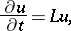(1)

where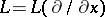is a differential operator,,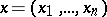, the absolutely stable implicit schemes of simple approximation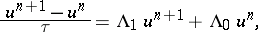(2)become ineffective in the case of multi-dimensional problems. In some cases a very small step in the unit of time is needed, in others finding each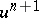requires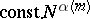operations, whereis the number of points per measurement,is the number of spatial measurements andstrongly increases asincreases.

For obtaining economical stable difference schemes methods are proposed based on the following ideas:

1) splitting of the difference schemes;

2) approximate factorization;

3) splitting (weak approximation) of the differential equations.

In the case of equation (1) the respective difference schemes have the following form (for the sake of simplicity, two fractional steps have been taken and the periodic Cauchy problem is considered):

the splitting scheme:(3)

the approximate factorization scheme:(4)the weak approximation scheme: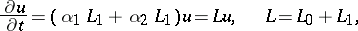(5)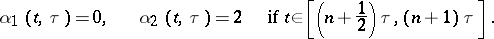In the case of the schemes (3) and (4) inversion of the operator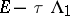is replaced by inversion of the operator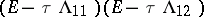, i.e. by successive inversion of the operators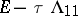,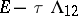, the structure of which is usually simpler.

Interpretation of (5) permits one to regard the splitting schemeas a simple approximation of equation (5) which weakly approximates equation (1).

Thus, these are methods based on the representation of complicated operators by simpler ones, and the integration of the initial equation is reduced to the integration of equations of a simpler structure, while the methods of fractional steps must satisfy the approximation and the stability conditions only in the final result (when written in "integral" steps). Many complicated problems in mathematical physics can be solved by the splitting method.

Splitting schemes of a high degree of accuracy have now attained a very advanced stage. One modification of this method is the so-called "particles-in-cells" method: The splitting is carried out according to physical processes and is independent of the reduction in the dimension of the operators.

How to Cite This Entry:
Fractional steps, method of. Encyclopedia of Mathematics. URL: http://encyclopediaofmath.org/index.php?title=Fractional_steps,_method_of&oldid=14759
This article was adapted from an original article by N.N. Yanenko (originator), which appeared in Encyclopedia of Mathematics - ISBN 1402006098. See original article